Advice: Solving equations involving numerical integration

Suppose you want to solve an equation involving a function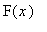, defined as a definite integral which Maple must evaluate numerically. For example, you might want to solve the equation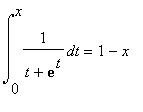. Using calculus, it is not hard to prove that this has exactly one solution with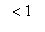. It is convenient to write the equation as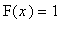:

> F:= x -> Int(1/(t + exp(t)), t=0..x) + x;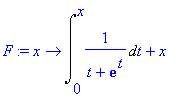We use the inert form Int rather than int , because int(1/(t+exp(t)), t=0..x) returns unevaluated. Now the following does not work:

> fsolve(F(x)=1, x = 0 .. 1);

Error, (in fsolve) should use exactly all the indeterminates

That is because of "premature evaluation": Maple first evaluates F(x) , obtaining an expression that includes the variable t , and fsolve objects to this. The usual cure for premature evaluation is to delay evaluation using quotes:

> fsolve('F(x)'=1, x = 0 .. 1);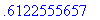This takes a rather long time to solve, since it requires evaluating the functionat many points, and each such evaluation requires another numerical integration. But another approach, based on differential equations, can be used. Note that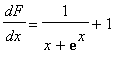, with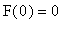. Now since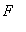is a one-to-one function of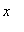, we can just as well consideras a function of. It will satisfy the differential equation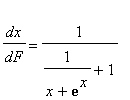with initial condition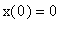, and what we want is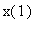.

> de:= diff(x(F),F) = 1/(1/(x(F)+exp(x(F)))+1);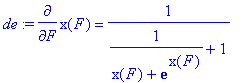> soln:= dsolve({de, x(0)=0},x(F), numeric);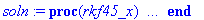> soln(1);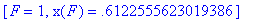This approach would not work in more general cases, e.g. an integral depending on a parameter whose value must be found. In those cases the solution must be found with fsolve .